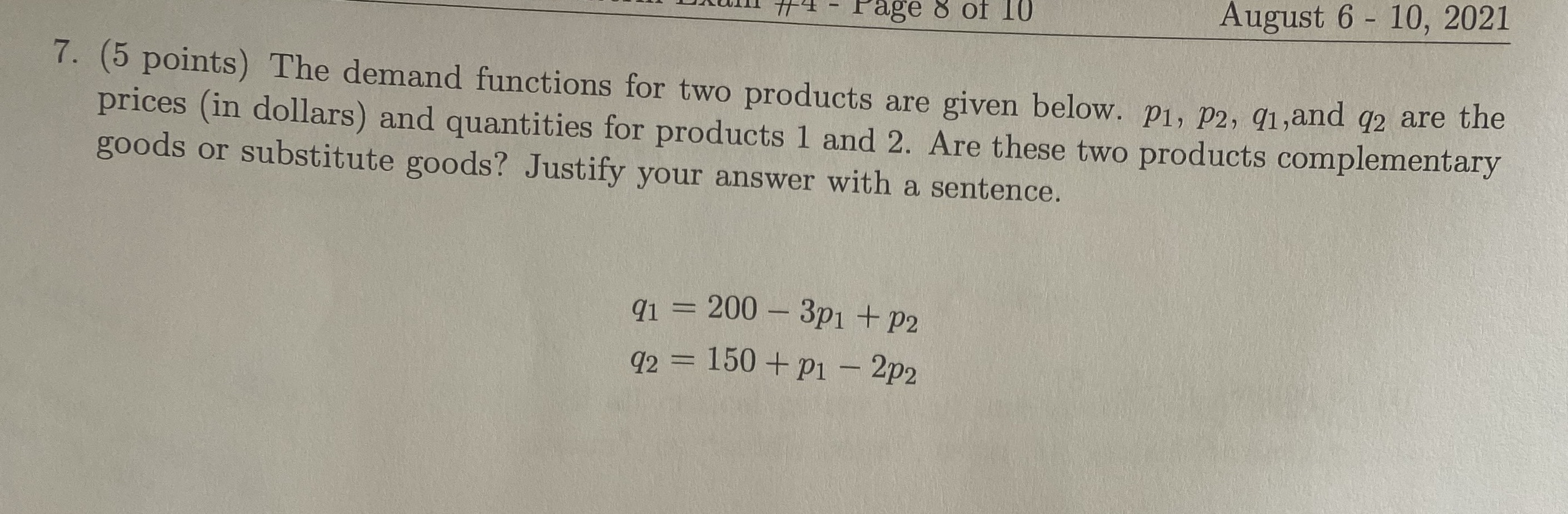### ¿Todavía tienes preguntas de matemáticas?

Pregunte a nuestros tutores expertos
Algebra
Pregunta(5 points) The demand functions for two products are given below. $$p _ { 1 } , p _ { 2 } , q _ { 1 } ,$$ and $$q _ { 2 }$$ are the prices (in dollars) and quantities for products $$1$$ and $$2$$ . Are these two products complementary goods or substitute goods? Justify your answer with a sentence.

$$q _ { 1 } = 200 - 3 p _ { 1 } + p _ { 2 }$$

$$q _ { 2 } = 150 + p _ { 1 } - 2 p _ { 2 }$$